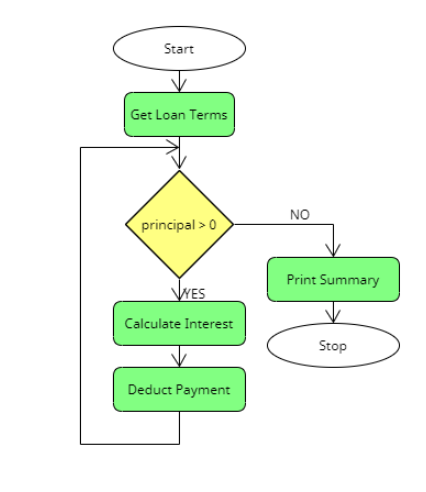$\require{cancel}$

# Flow of Control

• The flow of control is how a program process instructions.
• How do you follow a recipe?
• For programs:
• Usually there is some form of main statement where you start.
• Then you follow the instructions in order unless you encounter a control structure or a function call.
• get interest rate from the user
get principal from the user
get time from the user

interest = principal * rate * time
payment = principal + interest

print result 
• The above is pseudocode or informal high-level descriptions of algorithms and processes.
• Control structure:
• A statement that allows the programmer to change the flow of control.
• There are two basic types of control structures
• selection: if/if else
• called selection because we can "select" one path
• other structures in this class include case/switch
• // Convert the percent to a decimal
if interestRate > 1
interestRate = interestRate / 100
• Iteration
• Repeat a task until a goal is achieved
• repeat, for, while, do while ....
• while principal > 0
// deduct the monthly payment
principal = principal - monthlyPayment

// calculate one month interest
interest = principal*interestRate/12
principal = principal + interest
• Function calls
• Transfer control a different function.
• Functions are just a sequence of program instructions that perform a specific task, packages as a unit.
• Flow of control returns just after the calling point.
• get interest rate from the user
get principal from the user
get time from the user

Call Calculate Results
...
• Flowcharts
• I mostly do not use flowcharts.
• A flowchart is a diagram that represents an algorithm or process, showing steps as boxes connected by arrows which establish an order of flow.
• In a flowchart
• A oval is a start/stop of the logic
• A rectangle represents a task.
• A triangle represents a decision.
•• There are numerous tools for producing a flowchart.
• I used umlentino
• UML Common Elements has everyone you need.